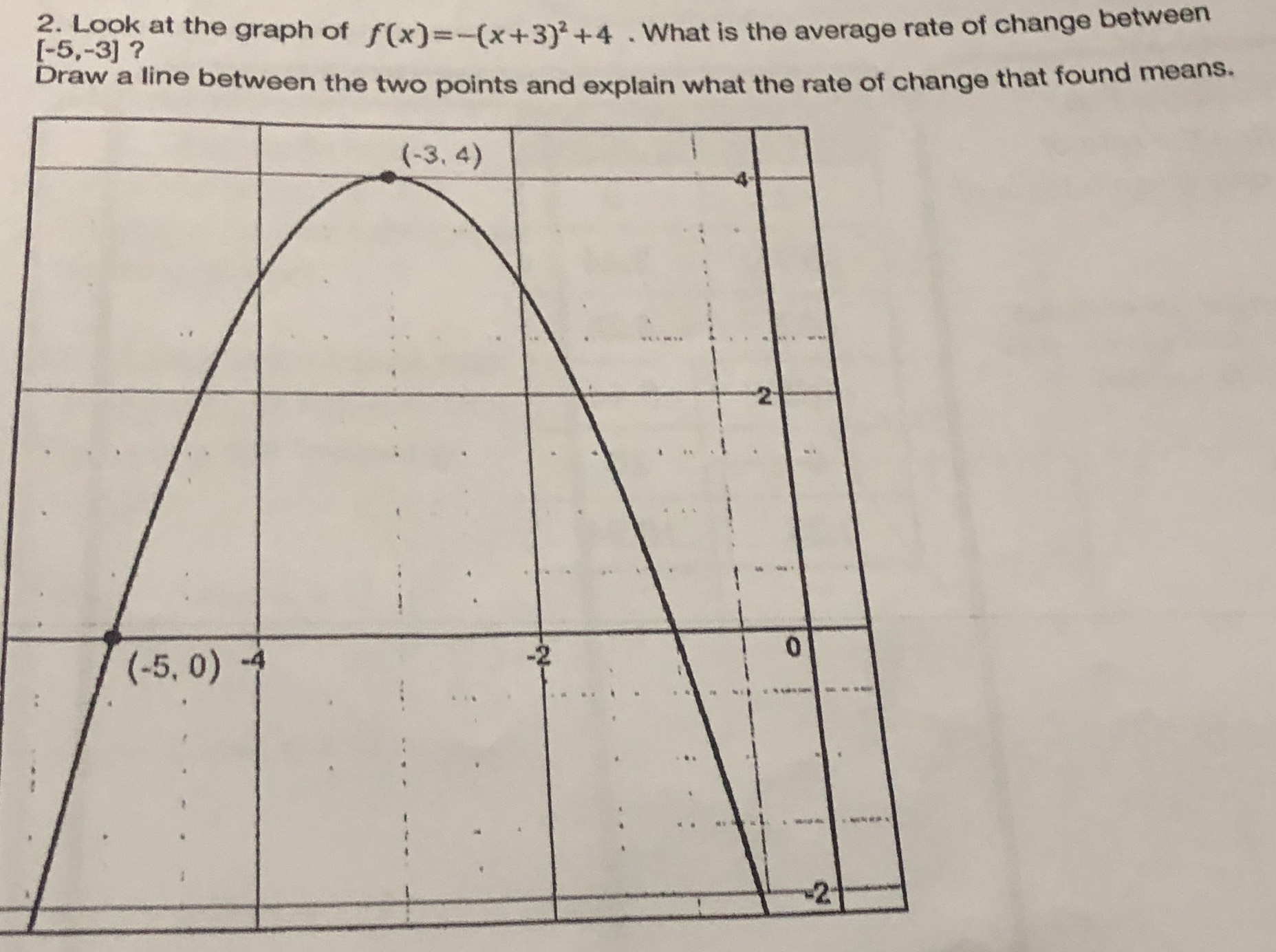### ¿Todavía tienes preguntas de matemáticas?

Pregunte a nuestros tutores expertos
Algebra
Pregunta2. Look at the graph of $$f ( x ) = - ( x + 3 ) ^ { 2 } + 4$$ . What is the average rate of change between $$[ - 5 , - 3 ]$$ ? Draw a line between the two points and explain what the rate of change that found means.

average rate of change = $$\frac{4- 0}{- 3+ 5} = 2$$